RS Aggarwal Class 7 Solutions Chapter 16 - Congruence

RS Aggarwal Class 7 Chapter 16 - Congruence Solutions Free PDF

When two objects or figures have the exact same shape and size, they are said to be congruent to each other. It is an important concept introduced in the topic of geometry. They can be similar in shape and size and also be mirror images of each other. The property of congruence is applicable for different shapes such as triangles, spheres, conic sections, polyhedra etc. Two sets of points can be congruent with each other and transformed on the basis of isometry especially reflection, translation and rotation.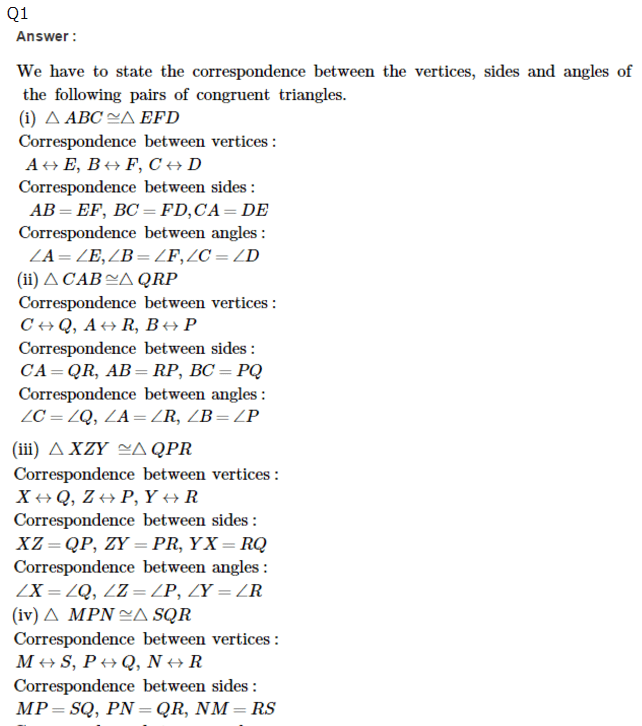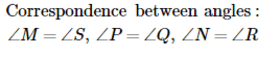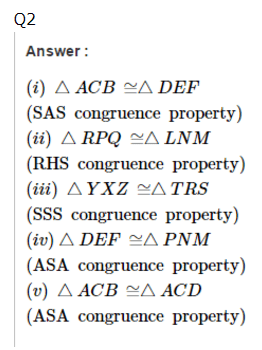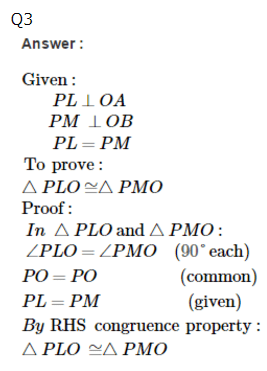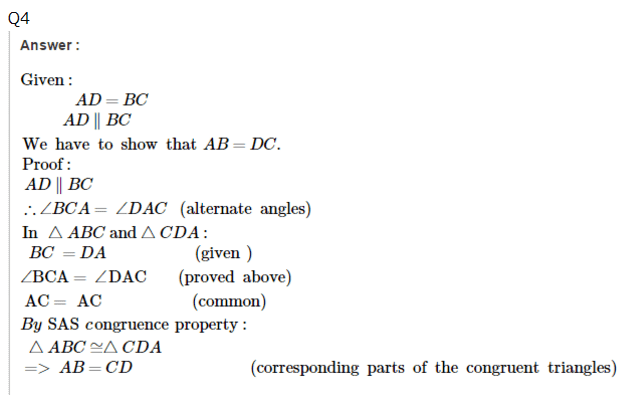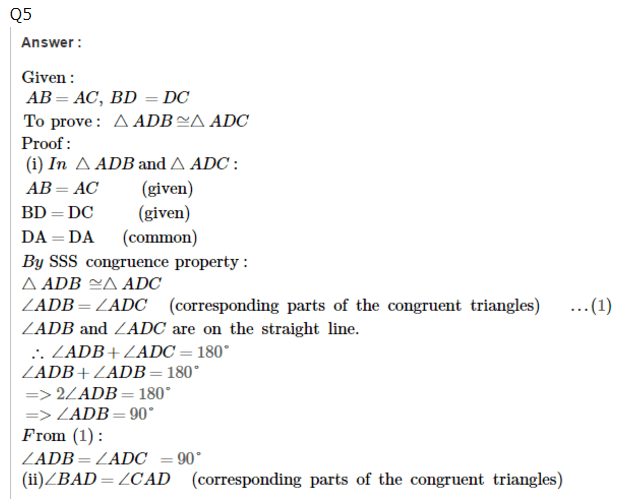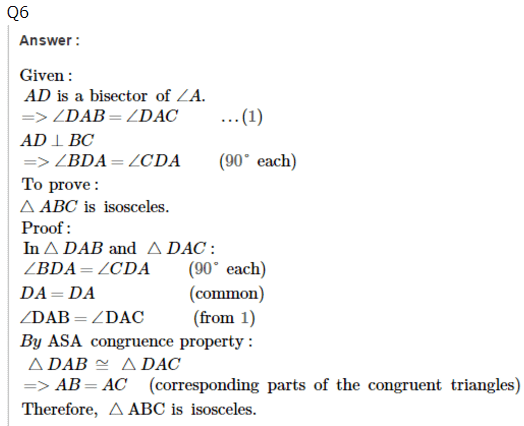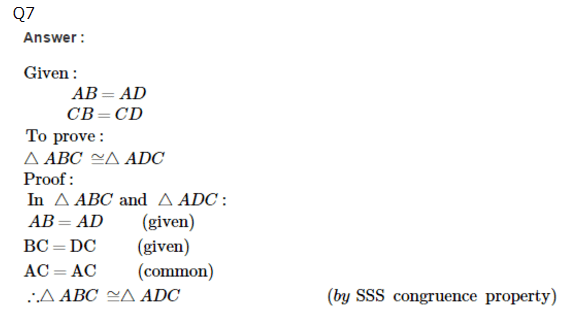Practise This Question

In Δ PQR PQ = 4 cm, QR = 7 cm and PR = 5 cm, then the largest angle of the triangle is: